# E-PolyLearning

 11. A centrifugal pump is used to pump water through a horizontal distance of 150 m, and then raised to an overhead tank 10 m above. The pipe is smooth with an I.D of 50 mm. What head (m of water) must the pump generate at its exit (E) to deliver water at a flow rate of 0.001 m³/s? The Fanning friction factor, f is 0.0062.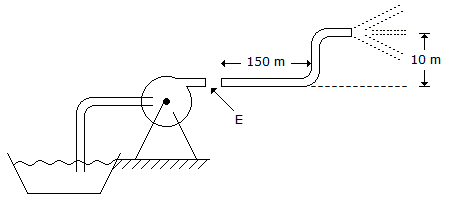a. 10 m b. 11 m c. 12 m d. 20 m

 12. Pick out the Kozney-Karman equation (valid for low Nʀₑ) for fluid flow through a packed bed of solids.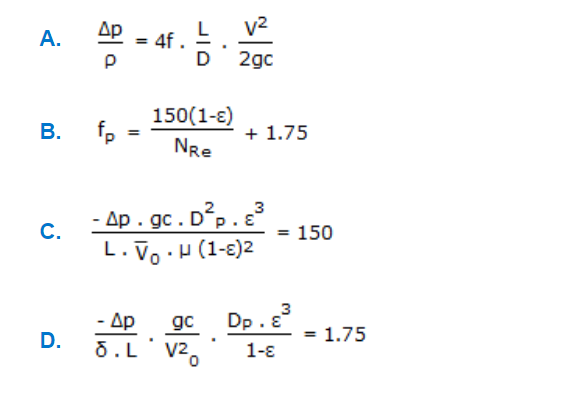a. A b. B c. C d. D
 13. Characteristic curves for a centrifugal pump plotted against its capacity is shown in the diagram. x, y and z denote respectively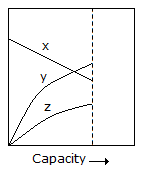a. efficiency, head and B.H.P. b. head, efficiency and B.H.P. c. B.H.P., efficiency and head d. efficiency, B.H.P. and head
 14. Specific speed for a centrifugal pump is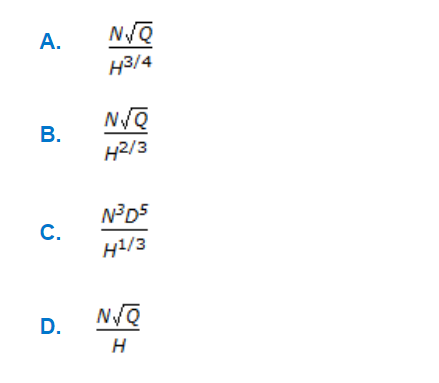a. A b. B c. C d. D
 15. For flow through a venturi at a particular discharge, the correct relationships among velocities at point X, Y and Z would be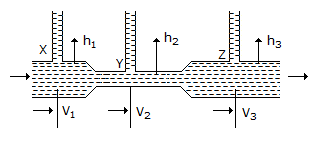a. V₁ < V₂ < V₃ b. V₂ > V₁ and V₂ > V₃ c. V₁ > V₂ > V₃ d. none of these.